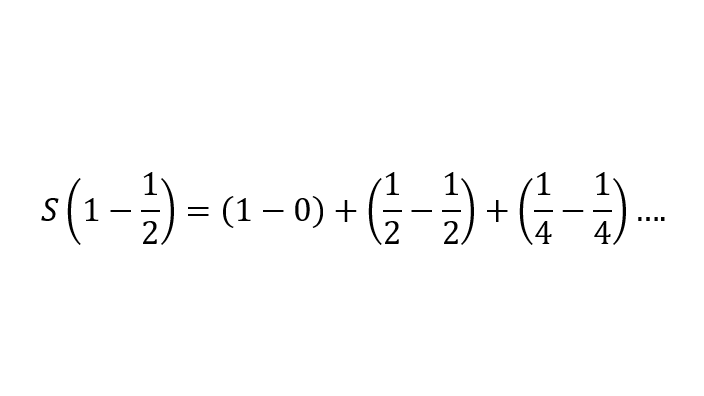top of page
Search

# A Paradox That Can Be Resolved [Review]

Updated: Mar 3, 2021

Author: Arpan DeyA fundamental question that confronts us is what do we mean by finite and infinite. In the early times, Zeno found the idea paradoxical that distance can be finite. For instance, let us assume a distance of two metres, from point A to point B. That is a finite distance, right? Or is it?

You could divide this distance into half, that is, go halfway from point A to B. But the distance from this point (the midpoint of A and B) to B can, still, be divided into half, and so on, infinitely…

This is Zeno’s dichotomy paradox. The paradox essentially addresses the question of whether every magnitude can be divided into half or not. If yes, as we must fundamentally assume, this would mean that no particle can ever reach its destination, say B from its starting point, say A. This is because, once half of the distance is covered, the portion that is left can again be divided into two equal parts… and so on. In fact, the argument proceeds by assuming that a particle needs to cover an infinite amount of distance to reach its destination, for no matter how tiny a portion of the journey is left, it can still be divided into two equal parts. We may know the actual distance from A to B, with respect to some system of measurements. However, the “actual distance” between A and B, that we measure, is less fundamental than the infinite series we obtain by continuously dividing the length into halves. This essentially means that only the first few terms of the infinite series are considerable, while the other terms are negligibly tiny, almost zero.

Mathematically, we can understand it in the following way. Let us say we have a distance of two units. We cut it down to half. We get a one-unit distance, and we will cut into further halves the other one-unit distance. Thus, we have 1 + 1. Dividing the second term into two halves, we get 1 + ½ + ½ . Dividing the last term into two equal parts again, we get 1 + ½ + ¼ + ¼ , and so on. Thus, we get an infinite series:Multiplying both sides by ½ , we get:Subtracting this expression from the previous one, we get:All other terms cancel out, to ultimately give 2. Thus, though the process is infinite, the result approaches 2 units, as is obvious. Thus, this paradox does not really challenge the existence of reality. However, it is still amazing that Zeno, so many years back, could think of this.

There are certain paradoxes which can be resolved. These paradoxes arise due to misinterpretation on our part, or lack of required knowledge. However, there undeniably remains paradoxes which are fundamental, and even puts in question our very existence.

An example of an incomplete paradox is this:

“This statement is false.”

If the above statement is true, then it is false. However, if it is false, then it must be true. Such so-called “paradoxes” are what I call “incomplete paradoxes.” Because, in the statement, “this” is referring to something else. As long as nothing definite is associated with the statement, the statement makes no sense.

A statement cannot be false and true at the same time – nor can a cat be both alive and dead at the same time. Or even if that is possible, in a different sense, we can never perceive it. The human mind fails to perceive ambiguity. For instance, in the picture below, you either perceive a duck or a rabbit; maybe the perception changes frequently. However, you cannot perceive both the duck and the rabbit at the same time.To quote Feynman,

“The “paradox” is only a conflict between reality and your feeling of what reality “ought to be”.”